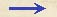Discorsi Propositions 2/13-pr-01 Discorsi Proposition2/13-pr-01PROBLEMA I, PROPOSITIO XIII. PROBLEM I, PROPOSITION XIIIDato perpendiculo, ad ipsum planum inflectere, in quo, cum ipsum habeat cum dato perpendiculo eandem elevationem, fiat motus post casum in perpendiculo eodem tempore, ac in eodem perpendiculo ex quiete. Given a perpendicular line of limited length, it is required to find a plane having a vertical height equal to the given perpendicular and so inclined that a body, having fallen from rest along the perpendicular, will make its descent along the inclined plane in the same time which it occupied in falling through the given perpendicular. Sit datum perpendiculum AB, cui, extenso in C, ponatur pars BC {10} aequalis, et ducantur horizontales CE, AG: oportet, ex B planum usque ad horizontem CE inflectere, in quo fiat motus post casum ex A eodem tempore, ac in AB ex quiete in A. Ponatur CD aequalis CB, et ducta BD, applicetur BE aequalis utrisque BD, DC: dico, BE esse planum quaesitum. Producatur EB, occurrens horizonti AG in G, et ipsarum EG, GB media sit GF; erit EF ad FB ut EG ad GF, et quadratum EF ad quadratum FB ut quadratum EG ad quadratum GF, hoc est {20} ut linea EG ad GB: est autem EG dupla GB: ergo quadratum EF duplum quadrati FB. Verum quadratum quoque DB duplum est quadrati BC; ergo ut linea EF ad FB, ita DB ad BC, et, componendo et permutando, ut EB ad duas DB, BC, ita BF ad BC: sed BE duabus DB, BC est aequalis: ergo BF ipsi BC, seu BA aequalis est. Si igitur intelligatur, AB esse tempus casus per AB, erit GB tempus per GB, et GF tempus per totam GE; ergo BF erit tempus per reliquam BE post casum ex G, seu ex A: quod erat propositum. Let AB denote the given perpendicular: prolong this line to C making BC equal to AB, and draw the horizontal lines CE and AG. It is required to draw a plane from B to the horizontal line CE such that after a body starting from rest at A has fallen through the distance AB, it will complete its path along this plane in an equal time. Lay off CD equal to BC, and draw the line BD. Construct the line BE equal to the sum of BD and DC; then, I say, BE is the required plane. Prolong EB till it intersects the horizontal AG at G. Let GF be a mean proportional between GE and GB; then EF:FB = EG:GF, and EF^2:FB^2= EG^2:GF^2 = EG:GB. But EG is twice GB; hence the square of EF is twice the square of FB; so also is the square of DB twice the square of BC. Consequently EF:FB = DB:BC, and componendo et permutando, EB:DB + BC = BF:BC. But EB = DB + BC; hence BF = BC = BA. If we agree that the length AB shall represent the time of fall along the line AB, (Condition 2/03-th-03-cor) then GB will represent the time of descent along GB, (Condition 2/02-th-02-cor2) and GF the time along the entire distance GE; (Condition 2/11-th-11) therefore BF will represent the time of descent along the difference of these paths, namely, BE, after fall from G or from A. Q. E. F.Discorsi Propositions 2/13-pr-01 Discorsi Proposition2/13-pr-01